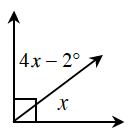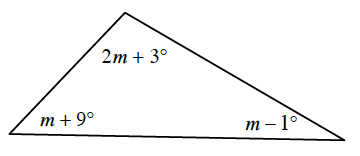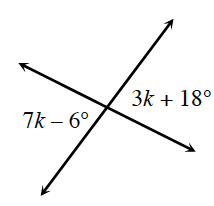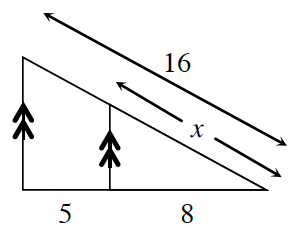Home > CCG > Chapter 6 > Lesson 6.2.4 > Problem6-84

6-84.

Examine the geometric relationships in each of the diagrams below. For each one, write and solve an equation to find the value of the variable. Name all geometric relationships or theorems that you use.

1.What is the sum of the measures of these angles?

$x=18.4º$

1.What is the sum of the measures of the angles of a triangle?

$m=42.25º$

1.How do these angles relate to each other?

1.These triangles are similar by $\text{AA}\sim$.
Since the triangles are similar, the ratios of corresponding sides are equal.

$x=9.8$